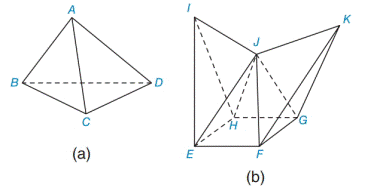Chapter 9.4, Problem 1EElementary Geometry For College St...

7th Edition
Alexander + 2 others
ISBN: 9781337614085

Solutions

Chapter
SectionElementary Geometry For College St...

7th Edition
Alexander + 2 others
ISBN: 9781337614085
Textbook Problem

Which of these two polyhedrons is concave? Note that the interior dihedral angle formed by the planes containing ∆EJF and ∆KJF is larger than 180 ° .To determine

To find:

The concave polyhedron.

Explanation

Approach:

1) A polygon is a two dimensional shape form with more than two straight lines.

2) A polyhedron is a three-dimensional shape made up of polygons as sides.

3) Each flat surface of a polyhedron is a polygon and is called a face. A polyhedron must have at least four faces.

4) The line segment where two faces of a polyhedron meet is called an edge.

5) The point where three or more edges of a polyhedron meet is called a vertex.

6) A dihedral angle is the angle between two faces with a common edge.

7) A polyhedron has dihedral angles between each of their sides because each side

of a polyhedron is a plane.

8) When a polyhedron is convex, each face determines a plane for which all remaining faces lie on same side of that plane...

Still sussing out bartleby?

Check out a sample textbook solution.

See a sample solution

The Solution to Your Study Problems

Bartleby provides explanations to thousands of textbook problems written by our experts, many with advanced degrees!

Get Started

Solve the equations in Exercises 126. (x+1)3+(x+1)5=0

Finite Mathematics and Applied Calculus (MindTap Course List)

The difference between population variance and sample variance.

Statistics for The Behavioral Sciences (MindTap Course List)

In Exercises 1520, simplify the expression. 20. 81x5y1039xy23

Applied Calculus for the Managerial, Life, and Social Sciences: A Brief Approach

In Exercises 1-8, determine which of the matrices are regular. 

Finite Mathematics for the Managerial, Life, and Social Sciences